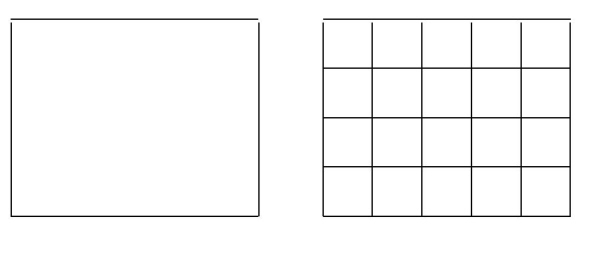# Infinity-categories vs Kan complexes

It is known (cf. Lurie's book Higher Topos Theory for instance) that higher ($\infty$-) category, in particular topological higher ($\infty$-) groupoids are "better" defined as weak Kan complexes, aka quasi-categories. Let me recall that a simplicial space $X_\bullet$ is weak Kan if every map $\Lambda_i^n\longrightarrow X$ (where $\Lambda_i^n$ is the horn) can be extended to a map $\Delta^n\longrightarrow X$ for all $0< i < n$.

My problem is the following. Let us consider the fundamental $(\infty,0)$-groupoid $\pi_{\leq \infty}X$ of a nice space $X$: $X$ is the set of objects, morphisms between objects are maps $f:[0,1]\longrightarrow X$, $2$-morphisms are homotopies between morphisms, and so on. I am quite confused about how does one, "geometrically", see $\pi_{\leq \infty}X$ as a simplicial space; for an element of $\pi_2X$, for instance, has four edges, and hence four different face maps.

• Your definition is that of an inner Kan complex, also known as a quasicategory, at least when $X$ is a simplicial set. Apr 26, 2013 at 17:48
• Just to clarify Zhen's comment: the definition of Kan complex in the question at the moment is not correct. A simplicial set (not space) is Kan if every horn (not just inner horns) can be filled. Apr 26, 2013 at 19:51
• Aside: while simplicial sets and cubical sets have been brought up, the specific model you're imagining for $\pi_{\leq \infty}(X)$ is a globular set.
– user13113
Dec 20, 2017 at 9:58

I suspect your confusion arises in part because homotopies of paths are continuous maps $I^2 \to X$, while 2-morphisms in $\pi_{\lt \infty} X$ are continuous maps $\Delta^2 \to X$. That is, 2-morphisms are not strictly the same as homotopies of paths.

The dictionary between the two structures is not too bad:

1. A 2-morphism in $\pi_{\lt \infty} X$ is a homotopy of paths, where either the beginning or the end is fixed (or perhaps it is a homotopy to or from a constant path).

2. A continuous map $I^2 \to X$ can be viewed as a composite of two 2-morphisms in $\pi_{\lt \infty} X$. You end up using the diagonal $(0,0) \to (1,1)$ in the square to separate the two 2-morphisms, because the simplicial structure of $\Delta^1 \times \Delta^1$ has a diagonal 1-simplex.

I personally find it rather magical that among the sixteen 2-simplices in $\Delta^1 \times \Delta^1$, a pair of them pops out as non-degenerate - it is a rewarding computation.

More generally, higher dimensional cubes, viewed as products of the simplicial set $\Delta^1$, have canonical decompositions into nondegenerate simplices. The corresponding higher homotopies are composites of homotopies with various pieces held constant.

I think this should account for the discrepancy you see in the number of face maps.

• Thanks, Carnahan, for this very interesting answer which, combined with Ronnie's intuitive bi-groupoid view point, helped me so much. Apr 28, 2013 at 19:23

To define the fundamental $\infty$-groupoid as a Kan complex, you should take your homotopies to live on simplices rather than cubes. The resulting object is also called the singular simplicial set. The $0$-simplices are points, and the $1$-simplices are paths as expected. But the $2$-simplices are maps from a $2$-simplex into $X$ with the expected number of face maps, etc.

• See Scott Carnahan's answer below for more about the relationship between the cubical and the simplicial way of thinking. Also, Ronnie Brown points out in his answer that the simplicial approach to higher groupoids is not necessarily "better", at least not in all regards. Apr 28, 2013 at 18:52

Recall that Kan's first paper was cubical, but subsequent papers were simplicial as difficulties were found with the standard cubical category, in particular that cubical groups were not Kan, and the realisation of the cartesian product of cubical sets did not have the homotopy type of the product of the realisations. These deficiencies have been overcome by using cubical sets with connections (as developed in work with Higgins) in papers by A. Tonks, (JPAA 81}~(1) (1992) 83--87) and G. Maltsiniotis, (HHA 11~(2) (2009) 309--326.) respectively.

In my own work with Higgins, we have used cubical sets extensively see the book Nonabelian algebraic topology: filtered spaces, crossed complexes, cubical homotopy groupoids. One major reason for the success of cubical methods is that they can easily express multiple compositions:Thus in the above diagram, you can ask non mathematicians if they think that there should be some mathematics in which the left hand square can be regarded as some kind of composition of the right hand square, and they will happily agree. Fortunately, this language exists, as double categories, and in the case of the cubical singular complex we can also write down such compositions as a composition $[a_{ij}]$ of an array $(a_{ij})$ of cubes. (See Remark 13.1.11 of the above book.) So we have an "algebraic inverse to subdivision" and this can be used to obtain new local-to-global results. The notion of "connections" on cubical sets arose in trying to define "commutative cubes".

The main theorems of the book were envisaged cubically, and eventually realised, but I feel that simplicially or globularly they would not even be thought of. Indeed, this programme was, what should I say, not welcomed by some.

Further, cubes are excellent for homotopies and higher homotopies, because of the rule $I^m \times I^n \cong I^{m+n}$.

So my answer to the question on $\pi_2 X$ and how to see it simplicially is that one should look to the geometry and not try to force an intuition into an uncomfortable mode, especially when a convenient and natural mode is available, or at any rate, the pros and cons should be thought about carefully.

• @jeq I use "square" in a space to be a map $a:I^2 \to X$ (and similarly for cube, $I^n \to X$) and we then consider $m \times n$ "subdivisions" of $a$. Oct 23, 2017 at 11:11